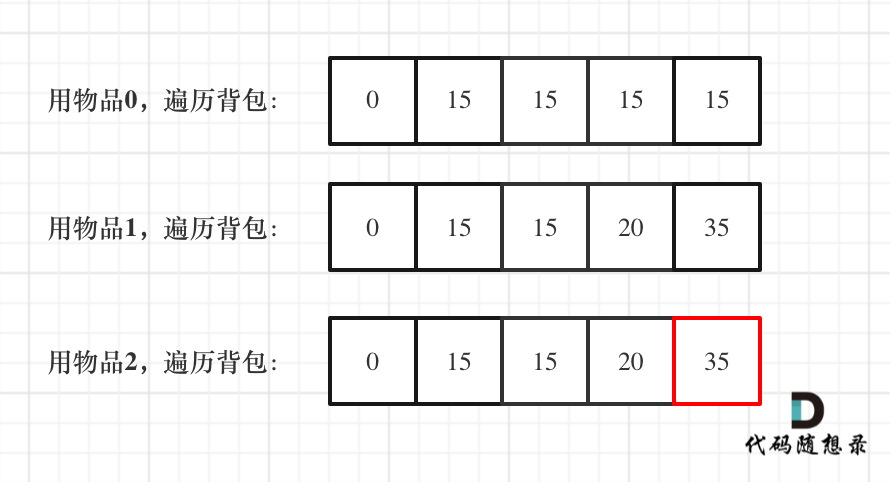# 4.01背包问题

  /**
* 0-1背包问题
* @param w w[index] 当前货物的总重量
* @param v v[index] 当前货物的总价值
* @param index 当前的货物号
* @param bag 可装的总重量
* @return 返回最大价值
*/

public static int process(int[] w,int[] v,int index,int alreadyW,int bag){
//base case 背高超重了，不能再放物品了
return -1;
}
//没有物品了
if(index==w.length){
return 0;
}
//当前物品不放所获得的最大价值
//当前物品放入背包所获得的最大价值
int pNext=-1;
if(p2!=-1){
pNext=v[index]+p2;
}
return Math.max(p1,pNext);
}


  /**
* 0-1背包问题
* @param w 当前货物的总重量
* @param v  当前货物的总价值
* @param index 当前的货物号
* @param rest 剩余可装入的重量
* @return 返回最大价值
*/

public static int process(int[] w,int[] v,int index,int rest) {
//没有容量了
if (rest == 0) {
return 0;
}
//没有物品了
if (index == w.length) {
return 0;
}
int p1 = process(w, v, index + 1, rest);
int p2 = 0;
//放入当前物品的话，要判段容量是否够
if (rest >= w[index]) {
p2 = v[index] + process(w, v, index + 1, rest - w[index]);
}

return Math.max(p1, p2);
}


    public static int bagDp(int[] w,int[] v,int rest){
int N=w.length;
int[][] dp=new int[N+1][rest+1];
for(int i=N-1;i>=0;i--){
for(int j=1;j<=rest;j++){
dp[i][j]=dp[i+1][j];
if(j-w[i]>=0){
dp[i][j]=Math.max(dp[i][j],dp[i+1][j-w[i]]+v[i]);
}
}
}
return dp[rest];
}


1. 确定dp数组以及下标的含义

dp[i][j] 表示从下标为[0-i]的物品里任意取，放进容量为j的背包，价值总和最大是多少。

1. 确定递推公式
• 不放物品i：由dp[i - 1][j]推出
• 放物品i：由dp[i - 1][j - weight[i]]推出，那么dp[i - 1][j - weight[i]] + value[i] （物品i的价值），就是背包放物品i得到的最大价值

1. dp数组如何初始化

1. 确定遍历顺序

1. 举例推导dp数组

 public static void testWeightBagProblem(int[] weight, int[] value, int bagSize){
int wLen = weight.length, value0 = 0;
//定义dp数组：dp[i][j]表示背包容量为j时，前i个物品能获得的最大价值
int[][] dp = new int[wLen + 1][bagSize + 1];
//初始化：背包容量为0时，能获得的价值都为0
for (int i = 0; i <= wLen; i++){
dp[i] = value0;
}
//遍历顺序：先遍历物品，再遍历背包容量
for (int i = 1; i <= wLen; i++){
for (int j = 1; j <= bagSize; j++){
if (j < weight[i - 1]){
dp[i][j] = dp[i - 1][j];
}else{
dp[i][j] = Math.max(dp[i - 1][j], dp[i - 1][j - weight[i - 1]] + value[i - 1]);
}
}
}
//打印dp数组
for (int i = 0; i <= wLen; i++){
for (int j = 0; j <= bagSize; j++){
System.out.print(dp[i][j] + " ");
}
System.out.print("\n");
}
}


## 一维dp数组（滚动数组）

1. 确定dp数组的定义

1. 一维dp数组的递推公式

dp[j] = max(dp[j], dp[j - weight[i]] + value[i]);

1. 一维dp数组如何初始化

dp[j]表示：容量为j的背包，所背的物品价值可以最大为dp[j]，那么dp就应该是0，因为背包容量为0所背的物品的最大价值就是0。

1. 一维dp数组遍历顺序
 public static void testWeightBagProblem(int[] weight, int[] value, int bagWeight){
int wLen = weight.length;
//定义dp数组：dp[j]表示背包容量为j时，能获得的最大价值
int[] dp = new int[bagWeight + 1];
//遍历顺序：先遍历物品，再遍历背包容量
for (int i = 0; i < wLen; i++){
for (int j = bagWeight; j >= weight[i]; j--){
dp[j] = Math.max(dp[j], dp[j - weight[i]] + value[i]);
}
}
//打印dp数组
for (int j = 0; j <= bagWeight; j++){
System.out.print(dp[j] + " ");
}
}


这里大家发现和二维dp的写法中，遍历背包的顺序是不一样的！

dp = dp[1 - weight] + value = 15

dp = dp[2 - weight] + value = 30

dp = dp[2 - weight] + value = 15 （dp数组已经都初始化为0）

dp = dp[1 - weight] + value = 15

（如何这里读不懂，大家就要动手试一试了，空想还是不靠谱的，实践出真知！）

（这里如果读不懂，就在回想一下dp[j]的定义，或者就把两个for循环顺序颠倒一下试试！）

1. 举例推导dp数组416. 分割等和子集

 public boolean canPartition(int[] nums) {
int sum=0;
for(int num:nums){
sum+=num;
}
int aim=sum/2;
if(sum%2!=0){
return false;
}
return process2dp(nums,aim);
}

private boolean process(int[] nums,int index,int aim){
if(index==nums.length){
return aim==0;
}

return process(nums,index+1,aim) ||
(aim-nums[index]>=0 && process(nums,index+1,aim-nums[index]));
}

private boolean process2dp(int[] nums,int aim){
// index 0---n  aim 0---aim
int n=nums.length;
boolean[][] dp=new boolean[n+1][aim+1];
dp[n]=true;
for(int i=n-1;i>=0;i--){
for(int j=0;j<=aim;j++){
dp[i][j]=dp[i+1][j];
if(j-nums[i]>=0){
dp[i][j] = dp[i][j] || dp[i+1][j-nums[i]];
}
}
}

return dp[aim];

}


#### 1049. 最后一块石头的重量 II

416. 分割等和子集 相当于是求背包是否正好装满，而本题是求背包最多能装多少。

class Solution {
public int lastStoneWeightII(int[] stones) {
int sum=0;
for(int s:stones){
sum+=s;
}
int target=sum>>1;
int res=process2dp(stones ,target);
return sum-2*res;

}
//151/2. =75.target.  151-2*dp[target]  求背包最多能装多少
// 31 26 21=78*2=156 33+40=73*2=146
//23  11. 11*2=22
//416. 分割等和子集 (opens new window)相当于是求背包是否正好装满，而本题是求背包最多能装多少。

//0--index  rest 最多能装多少
private int process(int[] stones,int index,int rest){
if(rest<=0){
return 0;
}
if(index==stones.length){
return 0;
}

int p1=  process(stones,index+1,rest);
int p2=Integer.MIN_VALUE;
if(rest-stones[index]>=0){
p2= process(stones,index+1,rest-stones[index])+stones[index];
}
return Math.max(p1,p2);
}

private int process2dp(int[] stones,int rest){
//N 0---N  rest 0---rest
int N=stones.length;
int[][] dp=new int[N+1][rest+1];
for(int i=N-1;i>=0;i--){
for(int j=1;j<=rest;j++){//注意这里的大于小于号不要写错了
int p1=dp[i+1][j];
int p2=Integer.MIN_VALUE;
if(j-stones[i]>=0){
p2=dp[i+1][j-stones[i]]+stones[i];
}
dp[i][j]=Math.max(p1,p2);
}
}
return dp[rest];
}

}


#### 494. 目标和

-1 + 1 + 1 + 1 + 1 = 3
+1 - 1 + 1 + 1 + 1 = 3
+1 + 1 - 1 + 1 + 1 = 3
+1 + 1 + 1 - 1 + 1 = 3
+1 + 1 + 1 + 1 - 1 = 3

public int findTargetSumWays(int[] nums, int target) {
return process(nums,0,0,target);
}

//可以转化为求和为0 ，a,b,c,d 每个数字都可以下负转化
private int process(int[] nums,int index,int sum,int target){
if(index==nums.length){
return sum==target?1:0;
}

return   process(nums,index+1,sum+nums[index],target)+
process(nums,index+1,sum-nums[index],target);
}


class Solution {
public int findTargetSumWays(int[] nums, int target) {
// l-r=target
// l+r=sum
// l-(sum-l)=target
//l=(sum+target)/2
//can find l in nums
//0...i  j dp[i][j]
int sum=0;
for(int num:nums){
sum+=num;
}
if((sum+target)%2!=0) return 0;
int size=(sum+target)/2;
if(size<0) size=-size;
return process2dp(nums,size);
}

public int process2dp(int[] nums,int size){
int[] dp=new int[size+1];
dp=1;
for(int i=0;i<nums.length;i++){
for(int j=size;j>=nums[i];j--){
dp[j]+=dp[j-nums[i]];
}
}
return dp[size];
}

}


• 1 <= strs.length <= 600
• 1 <= strs[i].length <= 100
• strs[i] 仅由 '0' 和 '1' 组成
• 1 <= m, n <= 100
class Solution {
int size=0;
public int findMaxForm(String[] strs, int m, int n) {
//  return process(strs,m,n,0,0,0);
return process2dp(strs,m,n);
}
// 01 001 100. index sum_1<=m sum_0<=n

private int process(String[] strs,int m,int n,int index,int sum_1,int sum_0){
if(index==strs.length){
return 0;
}

if(sum_0>m && sum_1>n){
return 0;
}

int p1= process(strs,m,n,index+1,sum_1,sum_0);
String s= strs[index];
int sum1=0;
int sum0=0;
for(char c:s.toCharArray()){
if(c=='0'){
sum0++;
}
if(c=='1'){
sum1++;
}

}
int p2=Integer.MIN_VALUE;
if(sum0+sum_0<=m && sum1+sum_1<=n){
p2= process(strs,m,n,index+1,sum_1+sum1,sum_0+sum0) + 1;
}
return Math.max(p1,p2);
}

private int process2dp(String[] strs,int m,int n){
//index 0---strs.length().  sum0. 0---m sum1 0---n
int N=strs.length;
int[][][] dp=new int[N+1][m+1][n+1];
for(int i=N-1;i>=0;i--){
for(int j=0;j<=m;j++){
for(int k=0;k<=n;k++){
int p1=dp[i+1][j][k];
String s= strs[i];
int sum1=0;
int sum0=0;
for(char c:s.toCharArray()){
if(c=='0'){
sum0++;
}
if(c=='1'){
sum1++;
}

}
int p2=Integer.MIN_VALUE;
if(sum0+j<=m && sum1+k<=n){
p2= dp[i+1][sum0+j][sum1+k]+1;
}

dp[i][j][k]=Math.max(p1,p2);
}
}
}

return dp;
}
}


posted @ 2021-10-30 08:55  sherry001  阅读(26)  评论(0编辑  收藏  举报